MORE IN Discrete Structures
SPPU Information Technology (Semester 3)
Discrete Structures
December 2014
Total marks: --
Total time: --
INSTRUCTIONS
(1) Assume appropriate data and state your reasons
(2) Marks are given to the right of every question
(3) Draw neat diagrams wherever necessary

Answer any one question from Q1 and Q2
1 (a) During a survey of the ice cream preferences of students, it was found that 22 like mango, 25 like custard apple, 39 like grape, 9 like custard apple and mango, 17 like mango and grape, 20 like custard apple and grape, 6 like all flavours and 4 like none. Then how many students were surveyed? How many students like exactly one flavour, how many students like exactly two flavours?
6 M
1 (b) What is recurrence relation ? Solve the following recurrence Relation:
ar-7ar-1+10ar-2=0
given that a0=0 and a1=3.
6 M

2 (a) State the principle of Mathematical Induction, using mathematical induction prove the following proposition: [ P(n)=1+4+7+ + \cdots \ + (3n-2) = \dfrac {n (3n-1)}{2}. ]
6 M
2 (b) Let A = {1, 2, 3, 4} and let R = {(1, 1), (1, 2), (1, 4), (2, 4), (3, 1), (3, 2), (4, 2), (4, 3), (4, 4)}. Find Transitive closure of R using Warshall's Algorithm.
6 M

Answer any one question from Q3 and Q4
3 (a) Consider an algebraic system (G, *), where G is the set of all non-zero real number and * is a binary operation defined by a * b = ab/4. Show that (G, *) is an Abelian Group.
6 M
3 (b) What do you understand by factors of a graph? Find all possible k-Factors of the following graph: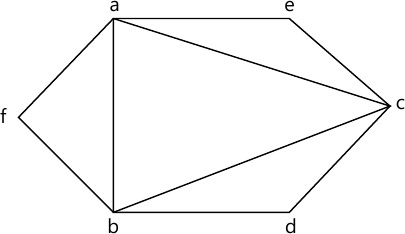6 M

4 (a) Find the degree of [f(x)+g(x)], [f(x)⋅g(x)] where the polynomials are on the integer (mod 8) and operations are addition and multiplication. You have f(x)=2x+4x2, g(x) = 2 + 6x + 4x2.
6 M
4 (b) Find the shortest path from a to z, using Dijkstra's Algorithm.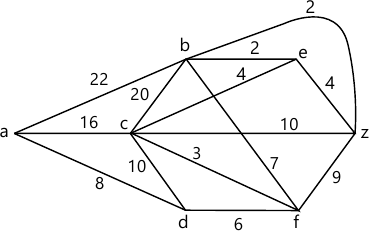6 M

Answer any one question from Q5 and Q6
5 (a) Determine the maximum flow in the following transport Network.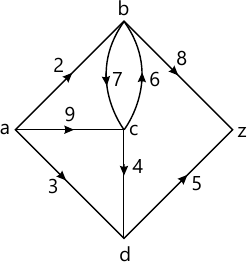7 M
5 (b) Find fundamental system of cut set for the graph G shown below with respect to the spanning tree T.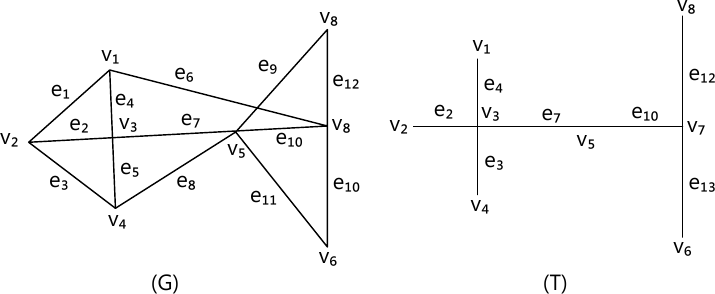6 M

6 (a) Define optimal tree. For the following set of weights, construct optimal binary prefix code. For each weight in the set, give corresponding code words ? 8, 9, 12, 14, 16, 19.
7 M
6 (b) Use the Kruskal's algorithm to find the minimum spanning tree for the graph shown in the figure.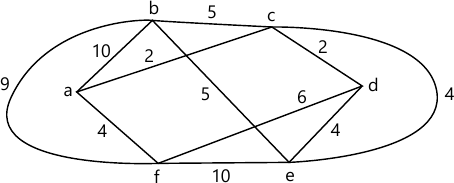6 M

Answer any one question from Q7 and Q8
7 (a) A single card is drawn from an ordinary deck S of 52 cards.
Find the probability p that:
i) The card is king.
ii) The card is a face card (jack, queen or king).
iii) The card is a heart.
iv) The card is a face.
6 M
7 (b) Find number of arrangement that can be made out of letters:
(i) ASSASSINATION
(ii) GANESHPURI.
7 M

8 (a) In a certain college town, 25% of the students failed in mathematics, 15% failed in chemistry, and 10% failed both in mathematics and chemistry. A student is selected at random:
(i) If he failed in chemistry, what is the probability that he failed in mathematics?
(ii) If he failed in mathematics, what is the probability that he failed in chemistry?
(iii) What is the probability that he failed in mathematics or chemistry?
(iv) What is the probability that he failed neither in mathematics nor in chemistry?
7 M
8 (b) 12 persons are made sit around a table. Find the number of ways they can sit such that 2 specific persons are not together.
6 M

More question papers from Discrete Structures